# Difference-set(2)

(diff) ← Older revision | Latest revision (diff) | Newer revision → (diff)

(update)

Letbe a group of order, and letbe a-subset of, such that the list of quotients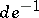with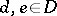contains each element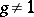exactlytimes. Thenis called a-difference set of order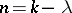in. Up to now (1998), only the case of Abelian difference sets (i.e.,is Abelian, cf. also Abelian difference set) has led to a satisfactory theory. The special case of cyclic difference sets is the one considered in Difference set (Vol. 3). For an extensive discussion of the theory of Abelian difference sets, see also [a1], Chap. VI.

The known families of difference sets can be subdivided into three classes:

difference sets with Singer parameters;

cyclotomic difference sets; and

difference sets with.

The difference sets with Singer parameters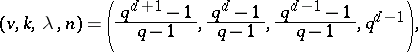whereis a prime power anda positive integer, include the classical Singer difference sets associated with the symmetric design formed by the points and hyperplanes of the projective space, the Gordon–Mills–Welch series and several infinite series for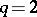, among them certain examples constructed from hyper-ovals (cf. also Oval) in Desarguesian projective planes of even order; see [a1], Sect. VI.17, and [a2]. The cyclotomic difference sets comprise the Paley difference sets, the families using higher-order residues and also the twin prime power series, see [a1], Sect. VI.8. The families of difference sets with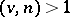are the Hadamard difference sets, the McFarland difference sets, the Spence difference sets with parameters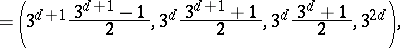whereis any positive integer, the Davis–Jedwab difference sets, with parameterswhereis a positive integer, and the Chen difference sets, with parameterswhere,is a prime number andis a positive integer; these were previously called generalized Hadamard difference sets and are known to exist wheneveris a power oforor the square of an odd prime power; see [a1], Sect. VI.9–12, for the construction of all these series.

The construction methods for the three general classes of difference sets are completely different: The Singer difference sets are cyclic and can be obtained from the action of a cyclic group of linear transformations on the one-dimensional subspaces of a finite field (viewed as a vector space over a suitable subfield), while the Gordon–Mills–Welch series is a "twisted" version of the Singer series and many of the remaining families with Singer parameters can be obtained from certain polynomials. Cyclotomic difference sets live in elementary Abelian groups (or the direct product of two such groups) and are unions of cosets of multiplicative subgroups of finite fields. The class of difference sets withis by far the richest; [a4] shows that all these difference sets are in fact very similar. [a4] also contains a recursive construction which covers all Abelian groups known to contain a difference set with(a modification is needed to include Chen's series, see [a1]). The best way to describe their construction is in terms of characters (cf. also Character of a group). The difference set is constructed from building blocks, smaller pieces which are in some sense orthogonal to each other with respect to the character group. This is based on the fact that the difference set condition can be translated into an equation in the integral group ring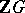; applying complex characters to the group ring element associated with, this translates into the condition that every non-trivial character yields a character sum of absolute value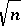; see [a1], Sect. VI.3. These character sums actually lie in the ring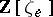of algebraic integers in the cyclotomic field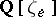(cf. also Cyclotomic polynomials), wheredenotes the exponent of(cf. Exponent of a group) and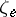is a primitiveth root of unity. This fact also allows the application of algebraic number theory (cf. also Algebraic number), which leads to strong non-existence results. Important progress with this approach was recently obtained by B. Schmidt [a8], see also [a1], Sect. VI.15–16. In particular, Schmidt obtained strong evidence for the validity of the long-standing circulant Hadamard matrix conjecture, which states the non-existence of a circulant Hadamard matrix of order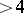and is equivalent to the perfect sequence conjecture; this also implies very strong evidence for the Barker sequence conjecture, which states the non-existence of a Barker sequence of length.

Cyclic difference sets are equivalent to binary periodic sequences with a-level autocorrelation function; that is, the periodic autocorrelation coefficients take on only one non-trivial value. Examples with particularly nice correlation properties (for instance,should be small in absolute value) have important applications in the engineering sciences, see [a6], [a7]. Some generalizations, e.g. Abelian relative difference sets, also yield interesting sequences, see [a6]. Similarly, difference sets in Abelian groups of rank(cf. also Rank of a group) correspond to periodic-dimensional arrays, which are likewise important in the engineering sciences, see [a7].

In recent years (1998), some progress regarding non-Abelian difference sets was obtained. Non-existence results follow from applying group representations, see e.g. [a5]. There are also some interesting constructions, see e.g. [a3].

How to Cite This Entry:
Difference-set(2). Encyclopedia of Mathematics. URL: http://encyclopediaofmath.org/index.php?title=Difference-set(2)&oldid=18522
This article was adapted from an original article by D. Jungnickel (originator), which appeared in Encyclopedia of Mathematics - ISBN 1402006098. See original article